# 直方图均衡化

## 引言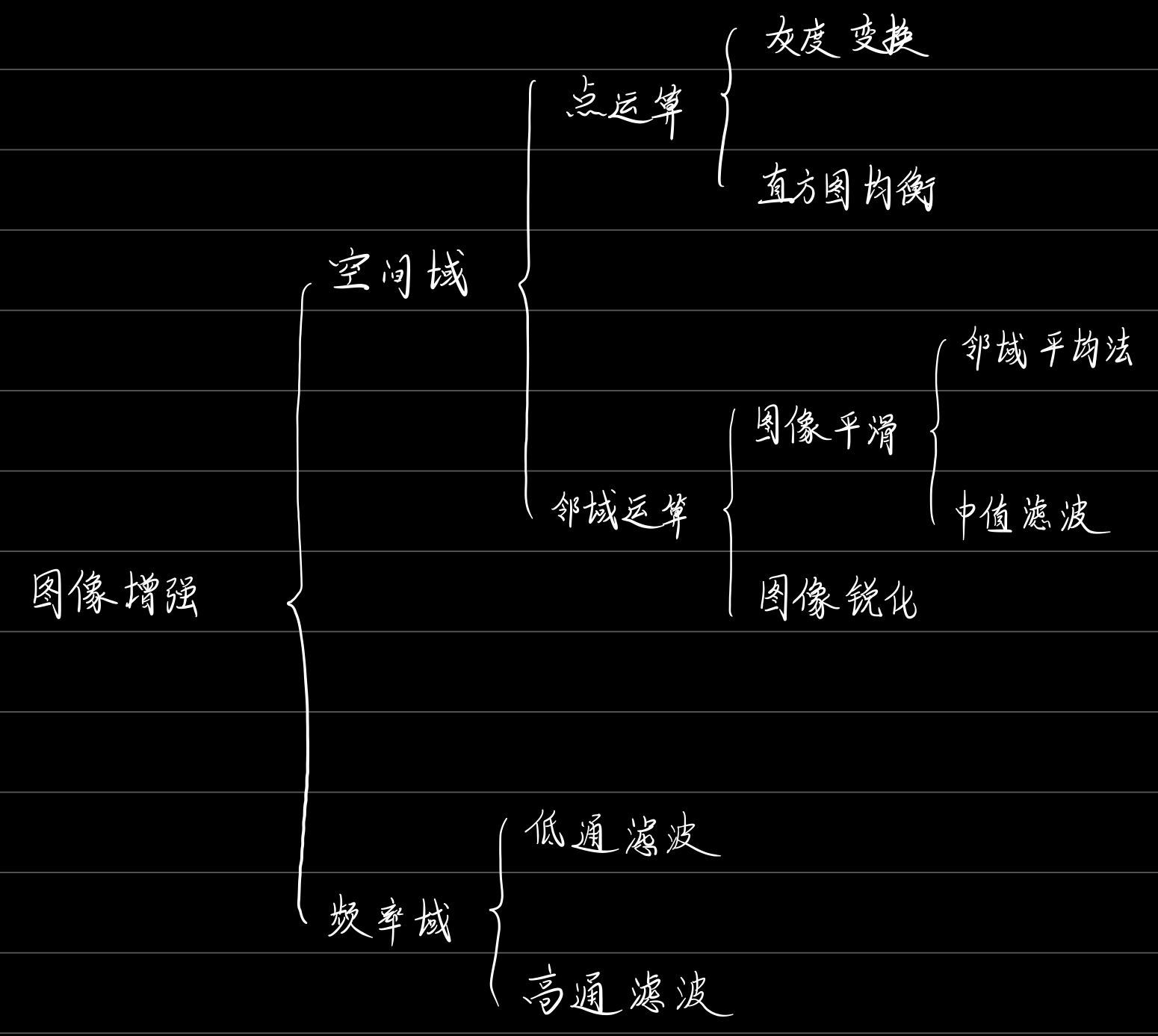## 直方图均衡化

### 直方图均衡化的概念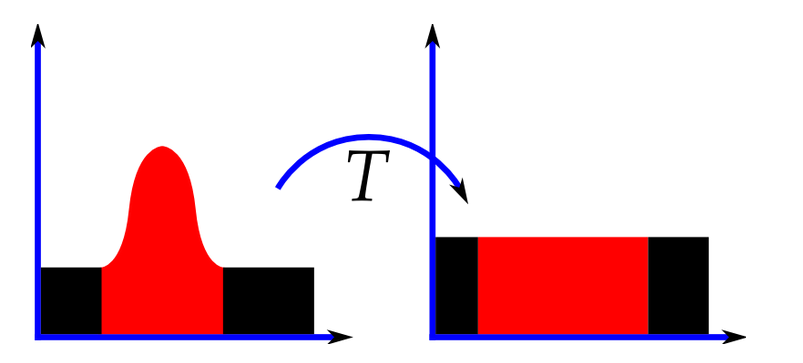S = T ( r ) = ( L − 1 ) ∫ 0 r p r ( w ) d w S=T(r)=(L-1)\int_0^rp_r(w)dw

S = T ( r ) ， 0 ≤ r ≤ L − 1 S=T(r)，0≤r≤L-1

T ( r ) T(r) 满足下列两个条件：
（1） T ( r ) T(r) 在区间 0 ≤ r ≤ L − 1 0≤r≤L-1 中为单调递增函数
（2）当 0 ≤ r ≤ L − 1 0≤r≤L-1 时， 0 ≤ T ( r ) ≤ L − 1 0≤T(r)≤L-1

• 条件（1）要求 T ( r ) T(r) 为单调递增函数是为了保证输出灰度值不少于相应的输出值，防止灰度反变换时产生人为缺陷。
• 条件（2）保证输出灰度的范围与输入灰度的范围相同

### 直方图均衡化的计算

P r ( r k ) = n k n , k = 0 , 1 , 2... , L − 1 P_r(r_k)= \frac{n_k}{n},k=0,1,2...,L-1

S k = T ( r k ) = ( L − 1 ) ∑ j = 0 k p r ( r j ) = ( L − 1 ) ∑ j = 0 k n j n k = 0 , 1 , . . . , 3 S_k=T(r_k)=(L-1)\sum_{j=0}^kp_r(r_j)=(L-1)\sum_{j=0}^{k}\frac{n_j}{n}\qquad k=0,1,...,3

### 简单举例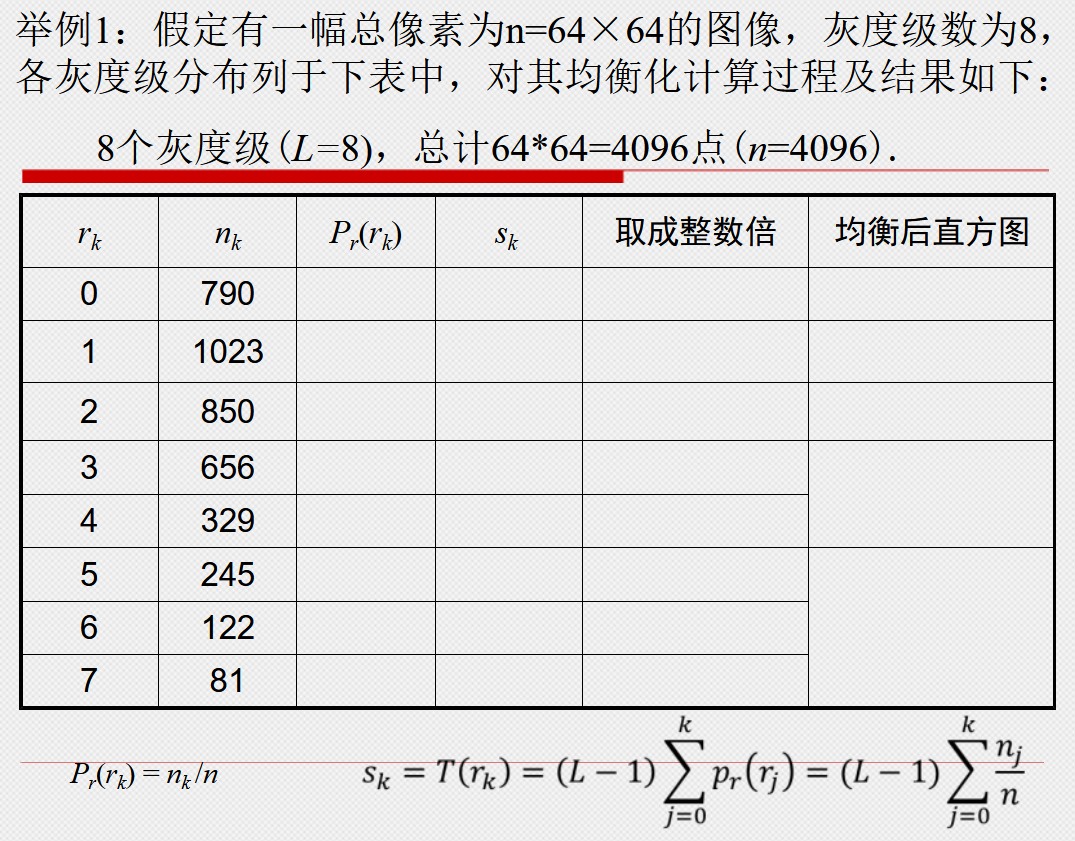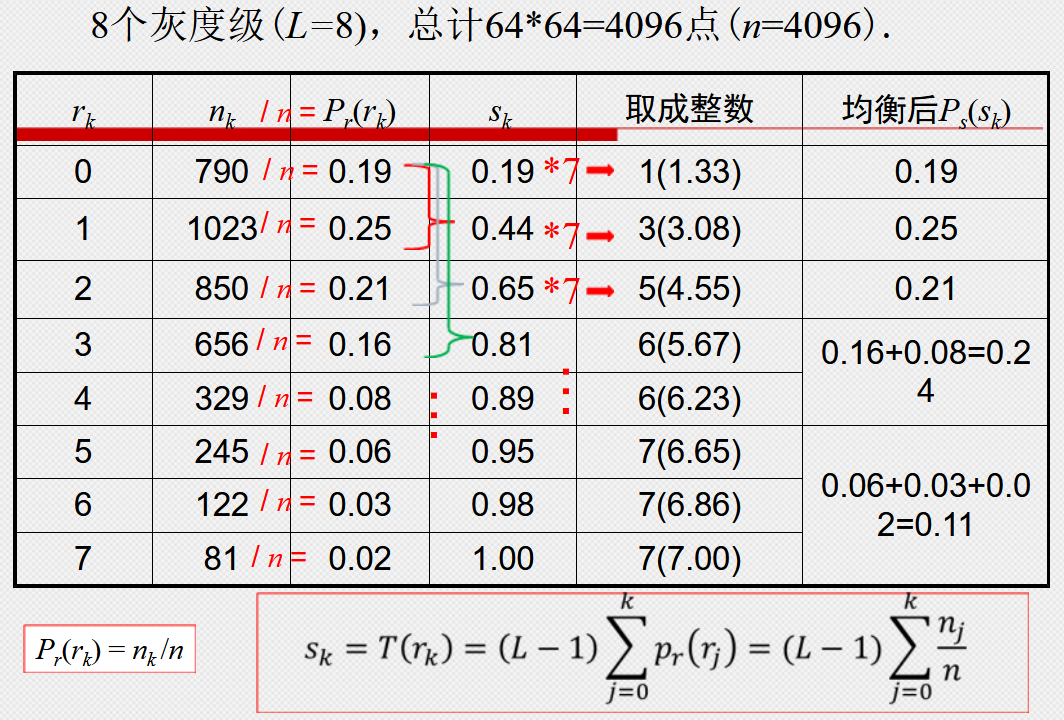# 自适应直方图均衡化(AHE)

## 简介

1、将图片均分成几片区域各自进行直方图均衡化；
2、对于一个特定像素，去其领域构建 S ( r ) S(r) 映射函数并将结果影射到该像素中；

# 限制对比度自适应直方图均衡化(CLAHE)

https://blog.csdn.net/u010839382/article/details/49584181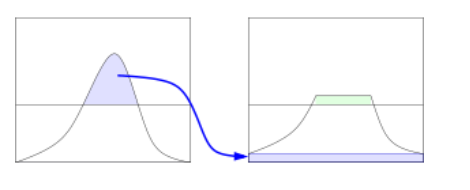matlab实现：

J = adapthisteq(I)03-02
11-22
07-272150
03-11
11-023万+
04-24113
07-19555
09-07245
04-081万+
03-05
10-105597
07-12876
11-083854
09-17303
03-14692
11-2365
03-211382
01-04515